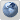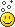# The Equations##The Equations

I have scoured Mathis' articles trying to extract the correct equations for gravity and E/M repulsion, but it is lost between the ongoing critiques of contemporary physics.

Has Mathis proposed a "attractive force due to gravity" equation, and a "repulsive force due to photon wind" that works at all scales and all bodies? I understand that the repulsive charge force for spinning particles would require an angle between the minima at polar and maxima at equator (sin θ?).

This is not from a lack of trying. I've spent many hours. I'm simply not clever enough to determine these equations. Providing them clearly would do wonders for everyone.

Last edited by jacksonc on Wed Nov 21, 2018 12:20 am; edited 1 time in total

##Re: The Equations

.

It’s my impression that Miles has well defined gravity - give or take an r^2 vice r^3 in the denominator. Miles identified the Unifield Field equations that break gravity and charge apart – at all scales.

If you’re trying to sift between conspiracy and science after several hours effort, you may not have been at Miles’ ‘mostly free from conspiracy’ Physics Homepage. THE GREATEST STANDING ERRORS IN PHYSICS AND MATHEMATICS by Miles Mathis, http://milesmathis.com/
All Miles' papers are organised by category in order to minimize such search efforts.

Again, welcome.  I certainly don't want to add to your frustration, let me know if his Homepage helps you or not.
.

LongtimeAirman

Posts : 1570
Join date : 2014-08-10

##Re: The Equations

Thanks. Indeed milesmathis.com was the home page I was scouring. With your reassurance I tried again. The closest thing to a definite equation for universal gravity was this:

a = 4mG /Δr2

So we calculate acceleration caused by the presence of a single body virtue of its mass and radius. I assume this radius refers to the radius of the gravitational body itself (not a distance). However, why is the radius expressed as a "change in radius"?

##Re: The Equations

What paper is that from? Some context for the equation will help to understand it and what it is useful for. Most equations in Physics have contextual requirements that limit the situations that you can apply the equation to. Hence a lack of general equations. Some are more general than others, but most still have some constraints on their applicability.

Since that equation only contains a single mass, it must be assuming all motion is applied to the other entity, and not the one denoted by the m variable. I assume the r represents the distance between their surfaces. Not sure why that would be marked as a delta though.Nevyn

Posts : 1882
Join date : 2014-09-11
Location : Australia##Re: The Equations

.
Yes, please be so kind as to list the paper source. I'm always happy to reread the appropriate papers before answering, but I can only read one or two papers at a time.

a = 4mG /Δr2. I assumed (correctly for once) that Δr is an expanding radius. I suppose you found that equation by way of #172.
172. http://milesmathis.com/third2.html The Third Wave: a Redefinition of Gravity, Part II. An explanation of both atomic and macro orbits using the expansion "field" and the E/M field. 7pp.

Let me direct you to another paper in which Miles derives that same equation based on the idea of expanding spheres.

19. http://milesmathis.com/ug.html The Universal Gravitational Constant. I follow a hint from Maxwell to give the dimensions of G to the mass. 6pp.

If that doesn't work, I'm sure we'll find some answer around here somewhere.
.

LongtimeAirman

Posts : 1570
Join date : 2014-08-10

##Re: The Equations

The paper I got this equation from is: /g.html (I'm not allowed to post external links).

Last edited by jacksonc on Mon Nov 19, 2018 11:18 pm; edited 1 time in total

##Re: The Equations

That equation does indeed represent all motion being applied to one of the entities and the reason it uses Δr is because it is actually using an expanding radius. This is an expansion equation and the radius is that of the particle, not the distance between 2 particles. We must assume that the particles are touching and one of the particles is being held still while the other is being pushed away as a result of the expansion. Therefore, a will tell you the acceleration of the particle being pushed.Nevyn

Posts : 1882
Join date : 2014-09-11
Location : Australia##Re: The Equations

Is Δr a function of time and G? I can't find this anywhere.

##Re: The Equations

To get to the crux of this question, what is the acceleration due to the charge field imposed upon a particle of radius r at distance d from the proton and at θ radians from the proton's equator?

##Re: The Equations

.
Is Δr a function of time and G? I can't find this anywhere.
Δr is based on ΔV and t, G is a transform between the photon and proton scales. 19. http://milesmathis.com/ug.html The Universal Gravitational Constant, goes into the details.

What is the acceleration due to the charge field imposed upon a particle of radius r at distance d from the proton and at θ radians from the proton's equator?
Your question confuses gravity and the charge field. The acceleration imposed upon a particle of radius r at distance d from the proton and at θ radians from the proton's equator is a description of the repulsive force of the charge field – not gravity.

The acceleration due to Gravity behaves exactly as though the proton (or the galaxy) were constantly expanding. As nearby protons expand, the distance between them appears to decrease, but actually the space between the two particles remains the same – it is being filled by the expanding particles themselves. Einstein’s famous thought experiment is slightly changed, one cannot tell the difference between the acceleration of the elevator through space, or the acceleration due to the elevator car sitting on the surface of an expanding planet.
.

LongtimeAirman

Posts : 1570
Join date : 2014-08-10

##Re: The Equations

That isn't a simple question to answer. It involves a few steps. Here is an outline:

1. Calculate distance from surface of emitter to surface of receiver.
2. Calculate angle from equator of emitter to receiver.
3. Calculate the charge density of the emitter at the equator of it's own surface.
4. Reduce the charge density based on the angle of receiver to emitter.
5. Calculate the charge density of the emitter at the receiver.Nevyn

Posts : 1882
Join date : 2014-09-11
Location : Australia##Re: The Equations

LongtimeAirman wrote:
Your question confuses gravity and the charge field. The acceleration imposed upon a particle of radius r at distance d from the proton and at θ radians from the proton's equator is a description of the repulsive force of the charge field – not gravity.

Sorry for confusion. I did indeed wish to know the repulsive force of the charge field as an equation if anyone knew of one.

##Re: The Equations

.
jacksonc wrote. "Has Mathis proposed a repulsive force due to gravity" equation, and a "repulsive force due to photon wind" that works at all scales and all bodies? I understand that the repulsive charge force for spinning particles would require an angle between the minima at polar and maxima at equator (sin θ?).
Your very first question at the top of the thread "Has Mathis proposed a repulsive force due to gravity", conflated/confused gravity and the charge field. There is no such thing as a repulsive force due to gravity. I thought you might still have that misunderstanding.

Gravity is strictly a function of a body's - (e.g. a proton) radius or surface velocity. It's important to note that gravitational expansion (along with pi = 4) may be the most difficult of all Miles' theories to accept. Many people reject the notion outright and the discussion could easily degenerate into something we're trying to avoid.

Determining the repulsive force on a particle due to the body's charge field emission field strength is fairly complicated as Nevyn indicated in his last post.
.

LongtimeAirman

Posts : 1570
Join date : 2014-08-10

##Re: The Equations

Just realized my mistake. I meant attractive force due to gravity and repulsive force due to charge. Whoops.##Re: The Equations